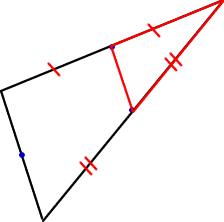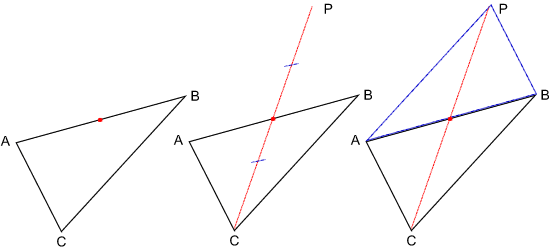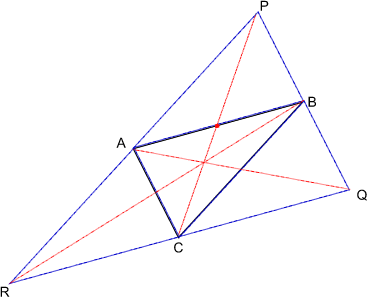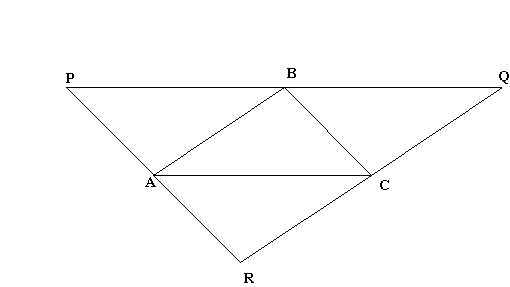#### You may also like### Fitting In

The largest square which fits into a circle is ABCD and EFGH is a square with G and H on the line CD and E and F on the circumference of the circle. Show that AB = 5EF. Similarly the largest equilateral triangle which fits into a circle is LMN and PQR is an equilateral triangle with P and Q on the line LM and R on the circumference of the circle. Show that LM = 3PQ### Look Before You Leap

Can you spot a cunning way to work out the missing length?Two ladders are propped up against facing walls. The end of the first ladder is 10 metres above the foot of the first wall. The end of the second ladder is 5 metres above the foot of the second wall. At what height do the ladders cross?

# Triangle Midpoints

##### Age 14 to 16Challenge Level

We received two different approaches to the solution. The first is based on what Stephanie from Beecroft School noticed.

Stephanie noticed that the large triangle is made from four congruent triangles, one of which is the triangle joining the midpoints. Stephanie therefore created the larger triangle by making three copies of the smaller one and translated and rotated them into place.

My question is:

How do you know the triangles are congruent? Does the following diagram help?Chip at King's Ely School and Eli sent in a solution based on Stephanie's ideas.

Take the three midpoints and call them A, B and C.
Draw the line between A and B and call it AB.
Take the midpoint of AB and measure the distance from it to C. Draw a line twice that distance from C through AB's midpoint. The end of that line is a corner of the original triangle, call it PRepeat this for the other corners forming the points Q and R which are the vertices of the required triangleLike my question above, I want to know how you know the vertices of the larger triangle can be found in this way? Also, if you can convince me you are right, how does the construction to find the midpoint of the sides of the triangle work and why?

The second approach involved parallel lines:

Call the mid points A, B and C Join A, B and C to form a triangle ABC
The original triangle PQR can be drawn by constructing a line through A parallel to BC, a line through B parallel to AC and a line through C parallel to AB.
You should then be able to prove that the points A, B and C are the mid-points of PR, PQ and QR.How do you know the sides of the larger triangle are parallel and how can you construct each of the lines parallel to the sides of the original triangle. Why does the construction work?